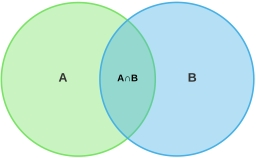# Sets

If X = {1, 2, 3, 4, 5 . . .10}, Y = {2, 4, 6,. . . 20} and Z = {x: x is an integer, 15 ≤ x ≤ 25 }.
Find (a) X ∩ Y, (b) X ∩ Z, (c) n(X ∪ Y) and (d) n(X ∪ Z)

a = \{2,4,5,6,8,10\}
b : \{\}=∅
c =  20
d =  25

### Step-by-step explanation:Did you find an error or inaccuracy? Feel free to write us. Thank you!